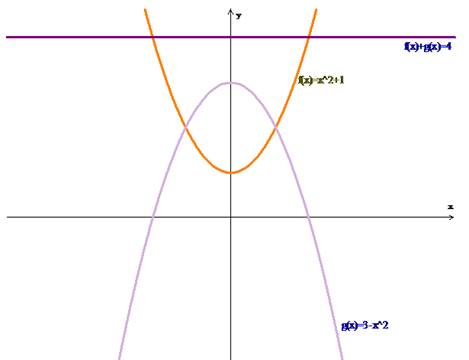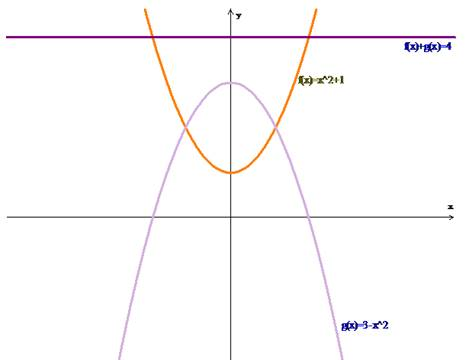# Draw the graphs of the functions f ( x ) , g ( x ) , f ( x ) + g ( x ) on the same graph.### Precalculus: Mathematics for Calcu...

6th Edition
Stewart + 5 others
Publisher: Cengage Learning
ISBN: 9780840068071### Precalculus: Mathematics for Calcu...

6th Edition
Stewart + 5 others
Publisher: Cengage Learning
ISBN: 9780840068071

#### Solutions

Chapter 2, Problem 74RE
To determine

## Draw the graphs of the functions f(x),g(x),f(x)+g(x) on the same graph.

Expert Solution

Graph of the function f(x),g(x)and f+g is shown below.### Explanation of Solution

Given information: Consider the functions f(x)=x2+1,g(x)=3x2

Calculation:

f(x)+g(x)=x2+1+3x2                   =4 This shows the graphical addition.

Thus, Graph of the function f(x),g(x)and f+g is shown below.### Have a homework question?

Subscribe to bartleby learn! Ask subject matter experts 30 homework questions each month. Plus, you’ll have access to millions of step-by-step textbook answers!